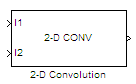# 2-D Convolution

Compute 2-D discrete convolution of two input matrices

• Library:
• Computer Vision Toolbox / Filtering

•## Description

The 2-D Convolution block computes the two-dimensional convolution of two input matrices. Assume that matrix A has dimensions (Ma, Na) and matrix B has dimensions (Mb, Nb). When the block calculates the full output size, the equation for the 2-D discrete convolution is:

`$C\left(i,j\right)=\sum _{m=0}^{\left(Ma-1\right)}\sum _{n=0}^{\left(Na-1\right)}A\left(m,n\right)*B\left(i-m,j-n\right)$`

where $0\le i and $0\le j.

## Ports

### Input

expand all

Input matrix, specified as either a matrix of intensity values or a matrix that represents one plane of an RGB video stream.

Data Types: `single` | `double` | `int8` | `int16` | `int32` | `int64` | `uint8` | `uint16` | `uint32` | `uint64` | `fixed point`

Input matrix, specified as either a matrix of intensity values or a matrix that represents one plane of an RGB video stream.

Data Types: `single` | `double` | `int8` | `int16` | `int32` | `int64` | `uint8` | `uint16` | `uint32` | `uint64` | `fixed point`

### Output

expand all

Convolution of the input matrices, returned as a matrix.

#### Dependencies

• The dimensions of the output are dictated by the Output size parameter.

• If the data type of the input is floating point, the output of the block is also floating point.

• If `all(size(I1)<size(I2))`, the block returns an error.

Data Types: `single` | `double` | `int8` | `int16` | `int32` | `int64` | `uint8` | `uint16` | `uint32` | `uint64` | `fixed point`

## Parameters

expand all

Main Tab

Dimensions of the output.

The table describes the block output, given the following input dimensions:

• I1 — (Ma, Na)

• I2 — (Mb, Nb)

Output sizeOutput Output Dimension
`Full`Full two-dimensional convolution(Ma+Mb-1, Na+Nb-1).
`Same as input port I1`Central part of the convolution with the same dimensions as the input at port I1
`Valid`Only the parts of the convolution that are computed without the zero-padded edges of any input.(Ma-Mb+1, Na-Nb+1)

Normalize output by dividing the output by `sqrt(sum(dot(I1p,I1p))*sum(dot(I2,I2)))`, where `I1p` is the portion of the I1 matrix that aligns with the I2 matrix.

### Note

When you select the Normalized output check box, the block input cannot be fixed point.

Data Types Tab

For details on the fixed-point block parameters, see Specify Fixed-Point Attributes for Blocks .

## Block Characteristics

 Data Types `double` | `fixed point` | `integer` | `single` Multidimensional Signals `no` Variable-Size Signals `yes`

expand all